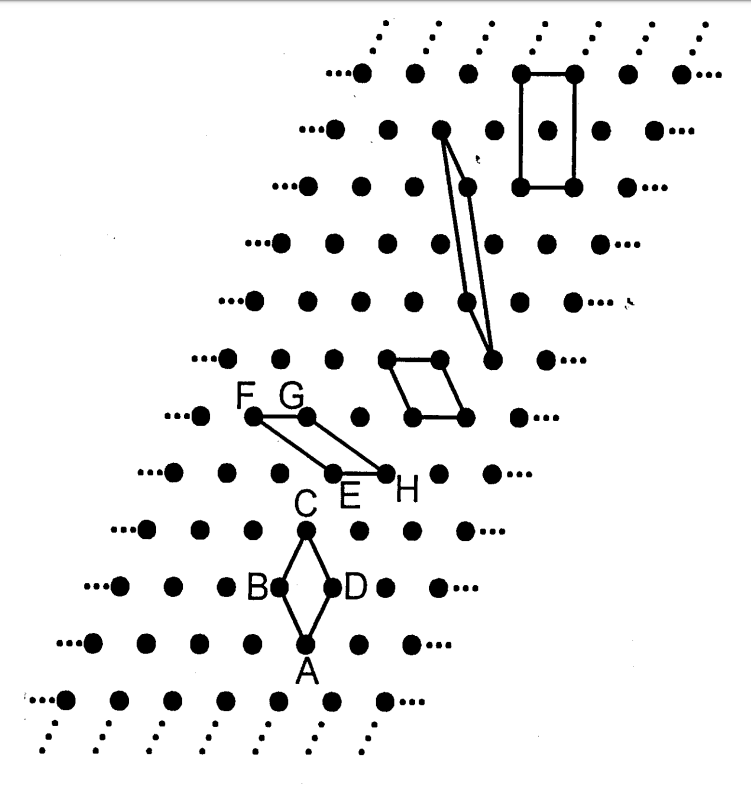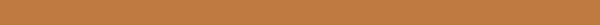Niggli reduce cell of 2D and 3D lattice

Code

https://github.com/unkcpz/pniggli

Introduction

Correct identification of the Bravais lattice of a crystal is an important early step in structure solution. Niggli reduction is a commonly used technique.

Examples in two dimensional lattice.An infinite variety of unit cells, vastly different in appearance and specification, can generate the same mathematical lattice.

Algorithm to determin Niggli cell(2D)

The following variables used in this algorithm are initialized for step A1-A4.

Define following variables as:

$$A=\bf{a\cdot a}$$ $$B=\bf{b\cdot b}$$ $$Y = \gamma = 2\bf{a\cdot b}$$

They are elements of metric tensor of $G$: $$G=\begin{pmatrix} A & \gamma/2 \\ \gamma/2 & B \\ \end{pmatrix}$$

$M$ represent the transformation matrix that is applied to basis vectors. The lattice vectors is represented as row vectors: $$L= \begin{pmatrix} \bf{a} \\ \bf{b} \end{pmatrix} = \begin{pmatrix} a_x & a_y \\ b_x & b_y \end{pmatrix}$$

$M$ operated as left transformation matrix to lattice vector $L$: $$\mathrm{new}\quad L = M \cdot L$$ that is, $$\begin{pmatrix} \bf{a'} \\ \bf{b'} \end{pmatrix} = M_{2\times 2} \cdot \begin{pmatrix} \bf{a} \\ \bf{b} \end{pmatrix}$$

Step 1

Ensure that basis length, $|\bf{a}| < |\bf{b}|$ that is $A < B$

If $A>B+eps$, let $(\bf{a'}, \bf{b'}) = (\bf{b}, \bf{a})$

$$M = \begin{pmatrix} 0 & 1 \\ 1 & 0 \end{pmatrix}$$

Step 2

Ensure that angle between $\bf{a}$ and $\bf{b}$ is obtuse:

If $Y > 0$, let $(\bf{a'}, \bf{b'}) = (\bf{a}, \bf{-b})$

$$M = \begin{pmatrix} 1 & 0 \\ 0 & -1 \end{pmatrix}$$

Step 3 and Step 4

Repeatedly overlapping basis $\bf{a}$ and $\bf{b}$ until that the angle between $\bf{a}$ and $\bf{b}$ reaches fullness.

Step 3:

If $abs(Y) > A + eps$, let $(\bf{a'}, \bf{b'}) = (\bf{a}, \bf{a+b})$

$$M = \begin{pmatrix} 1 & 0 \\ -sign(Y) & 1 \end{pmatrix}$$

Step 4:

If $abs(Y) > B + eps$, let $(\bf{a'}, \bf{b'}) = (\bf{a+b}, \bf{b})$

$$M = \begin{pmatrix} 1 & -sign(Y) \\ 0 & 1 \end{pmatrix}$$

Goto Step 1

Break the loop until A1-A4 are satisfied.

Algorithm to determin Niggli cell(3D)

The following variables used in this algorithm are initialized for step A1-A8.

Define following variables as:

$$A=\bf{a\cdot a}$$ $$B=\bf{b\cdot b}$$ $$C=\bf{c\cdot c}$$ $$X = \xi = 2\bf{a\cdot b}$$ $$E = \eta = 2\bf{c\cdot a}$$ $$Z = \zeta = 2\bf{b\cdot c}$$

They are elements of metric tensor of $G$: $$G=\begin{pmatrix} A & \xi/2 & \eta/2 \\ \xi/2 & B & \zeta/2 \\ \eta/2 & \xi/2 & C \end{pmatrix}$$

$M$ represent the transformation matrix that is applied to basis vectors. The lattice vectors is represented as row vectors: $$L= \begin{pmatrix} \bf{a} \\ \bf{b} \\ \bf{c} \end{pmatrix} = \begin{pmatrix} a_x & a_y & a_z \\ b_x & b_y & b_z \\ c_x & c_y & c_z \end{pmatrix}$$

$M$ operated as left transformation matrix to lattice vector $L$: $$\mathrm{new}\quad L = M \cdot L$$ that is, $$\begin{pmatrix} \bf{a'} \\ \bf{b'} \\ \bf{c'} \end{pmatrix} = M_{3\times 3} \cdot \begin{pmatrix} \bf{a} \\ \bf{b} \\ \bf{c} \end{pmatrix}$$

Step 1 and Step 2

Specification of the basis length sorting and basis angle sorting in G. Ensure that basis length, $|\bf{a}| < |\bf{b}| < |\bf{c}|$ that is

1. Ensure that basis length, $|\bf{a}| < |\bf{b}| < |\bf{c}|$, $A < B < C$,
2. and $abs(X) < abs(E) < abs(Z)$

If A > B + eps or (abs(A - B) < eps and abs(X) > abs(E) + eps) :

$$M = \begin{pmatrix} 0 & -1 & 0 \\ -1 & 0 & 0 \\ 0 & 0 & -1 \end{pmatrix}$$

Step 3 and step 4

Ensure that angle between $\bf{a}$ and $\bf{b}$ and $\bf{c}$ is obtuse:

$l, m, n$ represent angle type of $\xi, \eta, \zeta$.

• 1 for acute,
• -1 for obtuse
• and 0 for right respectively.

Step 3:

if $lmn=1$:

$$M = \begin{pmatrix} l & 0 & 0 \\ 0 & n & 0 \\ 0 & 0 & m \end{pmatrix}$$

Step 4:

Reference http://atztogo.github.io/niggli/#a4

If $l=-1, m=-1, n=-1$, do nothing.

If $lmn=0$ or $lmn=-1$:

Set $i=j=k=1$. $r$ is used as a reference to $i, j, \mathrm{or} k$, and is initially undefined.

• $i=-1$ if $l=1$
• $r\rightarrow i$ if $i=0$
• $j=-1$ if $m=1$
• $r\rightarrow j$ if $j=0$
• $k=-1$ if $n=1$
• $r\rightarrow k$ if $k=0$

if $ijk=-1$:

• $i, j, k$ refered by $r$ is set to -1.

$$M = \begin{pmatrix} i & 0 & 0 \\ 0 & j & 0 \\ 0 & 0 & k \end{pmatrix}$$

Step 5, 6 and 7

Repeatedly overlapping basis $\bf{a}$ and $\bf{b}$ until that the angle between $\bf{a}$ and $\bf{b}$ reaches fullness.

Step 5:

if (
abs(X) > B + eps
or (abs(X - B) < eps and 2 * E < Z - eps)
or (abs(X + B) < eps and Z < -eps)
):

$$M = \begin{pmatrix} 1 & 0 & 0 \\ 0 & 1 & 0 \\ 0 & -sign(X) & 1 \end{pmatrix}$$

Step 6:

if (
abs(E) > A + eps
or (abs(A - E) < eps and 2 * X < Z - eps)
or (abs(A + E) < eps and Z < -eps)
):

$$M = \begin{pmatrix} 1 & 0 & 0 \\ 0 & 1 & 0 \\ -sign(E) & 0 & 1 \end{pmatrix}$$

Step 7

if ( abs(X) > B + eps
or (abs(X - B) < eps and 2 * E < Z - eps)
or (abs(X + B) < eps and Z < -eps)
):

$$M = \begin{pmatrix} 1 & 0 & 0 \\ -sign(Z) & 1 & 0 \\ 0 & 0 & 1 \end{pmatrix}$$

Step 8

Get the basis metric element $C$ use $A$ and $B$.

If $\xi+\eta+\zeta+A+B<-\varepsilon$ or $abs(\xi+\eta+\zeta+A+B)<\varepsilon<\zeta+2(A+\eta)$

$$M = \begin{pmatrix} 1 & 0 & 0 \\ 0 & 1 & 0 \\ 1 & 1 & 1 \end{pmatrix}$$

Goto Step 1

Break the loop until A1-A4 are satisfied.

Reference

1. A Unified Algorithm for Determining the Reduced (Niggli) Cell, I. Krivý and B. Gruber, Acta Cryst., A32, 297-298 (1976)
2. The Relationship between Reduced Cells in a General Bravais lattice, B. Gruber, Acta Cryst., A29, 433-440 (1973)
3. Numerically stable algorithms for the computation of reduced unit cells, R. W. Grosse-Kunstleve, N. K. Sauter and P. D. Adams, Acta Cryst., A60, 1-6 (2004)
4. The geometry of Niggli reduction: BGAOL – embedding Niggli reduction and analysis of boundaries. Erratum . Journal of Applied Crystallography, 47(4), 1477–1477. Andrews, L. C., & Bernstein, H. J. (2014).
5. Selling reduction versus Niggli reduction for crystallographic lattices. Acta Crystallographica Section A: Foundations and Advances, 75(1), 115–120. Andrews, L. C., Bernstein, H. J., & Sauter, N. K. (2019).
6. https://nvlpubs.nist.gov/nistpubs/sp958-lide/188-190.pdf